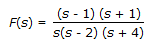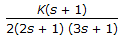# Chemical Engineering - Process Control and Instrumentation

### Exercise :: Process Control and Instrumentation - Section 7

16.

A control system has the following transfer functionThe initial value of the corresponding time function is

 A. 1 B. 1/8 C. 7/8 D. -1

Explanation:

No answer description available for this question. Let us discuss.

17.

For measuring the temperature of a red hot furnace, which is the most suitable instrument ?

 A. Platinum resistance thermometer B. Thermocouple C. Optical pyrometer D. Bimetallic thermometer

Explanation:

No answer description available for this question. Let us discuss.

18.

Continuous measurement of specific gravity of a liquid is done by

 A. hydrometer B. contact-type electric indicators C. displacement meter D. both (a) and (c)

Explanation:

No answer description available for this question. Let us discuss.

19.

Stroboscope is used for the measurement of

 A. rpm of a flywheel B. frequency of light C. depression of freezing point D. liquid level under pressure

Explanation:

No answer description available for this question. Let us discuss.

20.

The root locus plot of the roots of the characteristics equation of a closed loop system having the open loop transfer functionwill have a definite num-ber of loci for variation of K from 0 to ∞. The number of loci is

 A. 1 B. 3 C. 4 D. 2